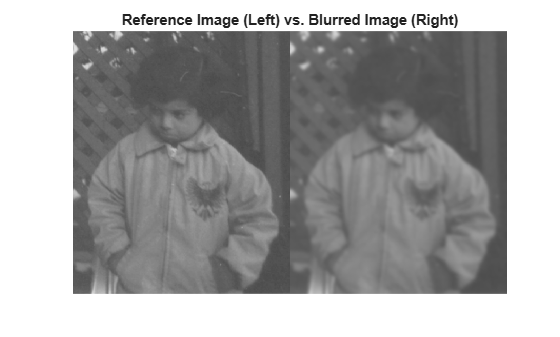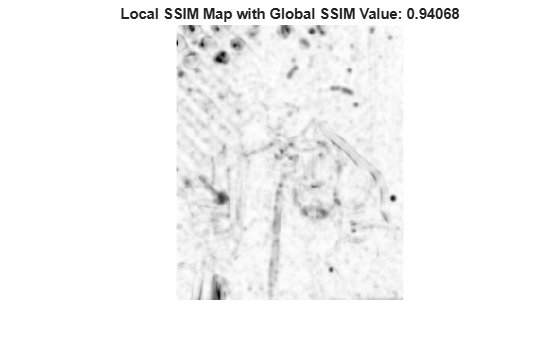Main Content

# ssim

Structural similarity (SSIM) index for measuring image quality

## Syntax

``ssimval = ssim(A,ref)``
``````[ssimval,ssimmap] = ssim(A,ref)``````
``___ = ssim(A,ref,Name,Value)``

## Description

````ssimval = ssim(A,ref)` calculates the structural similarity (SSIM) index for grayscale image or volume `A` using `ref` as the reference image or volume.```

example

``````[ssimval,ssimmap] = ssim(A,ref)``` also returns the local SSIM value for each pixel or voxel in `A`. ```
````___ = ssim(A,ref,Name,Value)` calculates the SSIM, using name-value pairs to control aspects of the computation.```

## Examples

collapse all

Read an image into the workspace. Create another version of the image, applying a blurring filter.

```ref = imread('pout.tif'); H = fspecial('Gaussian',[11 11],1.5); A = imfilter(ref,H,'replicate');```

Display both images as a montage. The images differ most along sharp high-contrast regions, such as the edges of the trellis.

```montage({ref,A}) title('Reference Image (Left) vs. Blurred Image (Right)')```Calculate the global SSIM value for the image and local SSIM values for each pixel.

`[ssimval,ssimmap] = ssim(A,ref);`

Display the local SSIM map. Include the global SSIM value in the figure title. Small values of local SSIM appear as dark pixels in the local SSIM map. Regions with small local SSIM value correspond to areas where the blurred image noticeably differs from the reference image. Large values of local SSIM value appear as bright pixels. Regions with large local SSIM correspond to uniform regions of the reference image, where blurring has less of an impact on the image.

```imshow(ssimmap,[]) title(['Local SSIM Map with Global SSIM Value: ',num2str(ssimval)])```## Input Arguments

collapse all

Image whose quality is to be measured, specified as a 2-D grayscale image or 3-D grayscale volume.

Data Types: `single` | `double` | `int16` | `uint8` | `uint16`

Reference image against which quality is measured, specified as a 2-D grayscale image or 3-D grayscale volume of the same size and data type as `A`

Data Types: `single` | `double` | `int16` | `uint8` | `uint16`

### Name-Value Pair Arguments

Specify optional comma-separated pairs of `Name,Value` arguments. `Name` is the argument name and `Value` is the corresponding value. `Name` must appear inside quotes. You can specify several name and value pair arguments in any order as `Name1,Value1,...,NameN,ValueN`.

Example: `ssim(A,ref,'DynamicRange',100)`

Dynamic range of the input image, specified as a positive scalar. The default value of `DynamicRange` depends on the data type of image `A`, and is calculated as `diff(getrangefromclass(A))`. For example, the default dynamic range is `255` for images of data type `uint8`, and the default is `1` for images of data type `double` or `single` with pixel values in the range [0, 1].

Data Types: `single` | `double` | `int8` | `int16` | `int32` | `uint8` | `uint16` | `uint32`

Exponents for the luminance, contrast, and structural terms, specified as a 3-element vector of nonnegative numbers of the form ```[alpha beta gamma]```.

Data Types: `single` | `double` | `int8` | `int16` | `int32` | `uint8` | `uint16` | `uint32`

Standard deviation of isotropic Gaussian function, specified as a positive number. This value is used for weighting the neighborhood pixels around a pixel for estimating local statistics. This weighting is used to avoid blocking artifacts in estimating local statistics.

Data Types: `single` | `double` | `int8` | `int16` | `int32` | `uint8` | `uint16` | `uint32`

Regularization constants for the luminance, contrast, and structural terms, specified as a 3-element vector of nonnegative numbers of the form `[c1 c2 c3]`. The `ssim` function uses these regularization constants to avoid instability for image regions where the local mean or standard deviation is close to zero. Therefore, small non-zero values should be used for these constants.

By default,

• `C1 = (0.01*L).^2`, where `L` is the specified `DynamicRange` value.

• `C2 = (0.03*L).^2`, where `L` is the specified `DynamicRange` value.

• `C3 = C2/2`

Data Types: `single` | `double` | `int8` | `int16` | `int32` | `uint8` | `uint16` | `uint32`

## Output Arguments

collapse all

SSIM index, returned as a numeric scalar. `ssimval` is of data type `double` except when `A` is of data type `single`, in which case `ssimval` is of data type `single`.

The value of `ssimval` is typically in the range [0, 1]. The value 1 indicates the highest quality and occurs when `A` and `ref` are equivalent. Smaller values correspond to poorer quality. For some combinations of inputs and name-value pair arguments, `ssimval` can be negative.

Local values of SSIM index, returned as a numeric array of nonnegative numbers of the same size as the input image, `A`. `ssimmap` is of data type `double` except when `A` is of data type `single`, in which case `ssimmap` is of data type `single`.

## More About

collapse all

### Structural Similarity Index

An image quality metric that assesses the visual impact of three characteristics of an image: luminance, contrast and structure.

## Algorithms

The SSIM Index quality assessment index is based on the computation of three terms, namely the luminance term, the contrast term and the structural term. The overall index is a multiplicative combination of the three terms.

`$SSIM\left(x,y\right)={\left[l\left(x,y\right)\right]}^{\alpha }\cdot {\left[c\left(x,y\right)\right]}^{\beta }\cdot {\left[s\left(x,y\right)\right]}^{\gamma }$`

where

`$\begin{array}{l}l\left(x,y\right)=\frac{2{\mu }_{x}{\mu }_{y}+{C}_{1}}{{\mu }_{x}^{2}+{\mu }_{y}^{2}+{C}_{1}},\\ c\left(x,y\right)=\frac{2{\sigma }_{x}{\sigma }_{y}+{C}_{2}}{{\sigma }_{x}^{2}+{\sigma }_{y}^{2}+{C}_{2}},\\ s\left(x,y\right)=\frac{{\sigma }_{xy}+{C}_{3}}{{\sigma }_{x}{\sigma }_{y}+{C}_{3}}\end{array}$`

where μx, μy, σxy, and σxy are the local means, standard deviations, and cross-covariance for images x, y. If α = β = γ = 1 (the default for `Exponents`), and C3 = C2/2 (default selection of C3) the index simplifies to:

`$SSIM\left(x,y\right)=\frac{\left(2{\mu }_{x}{\mu }_{y}+{C}_{1}\right)\left(2{\sigma }_{xy}+{C}_{2}\right)}{\left({\mu }_{x}^{2}+{\mu }_{y}^{2}+{C}_{1}\right)\left({\sigma }_{x}^{2}+{\sigma }_{y}^{2}+{C}_{2}\right)}$`

When you specify a noninteger value for '`Exponents`', the `ssim` function prevents complex valued outputs by clamping the intermediate luminance, contrast, and structural terms to the range [0, `inf`].

 Zhou, W., A. C. Bovik, H. R. Sheikh, and E. P. Simoncelli. "Image Qualifty Assessment: From Error Visibility to Structural Similarity." IEEE Transactions on Image Processing. Vol. 13, Issue 4, April 2004, pp. 600–612.

Download ebook